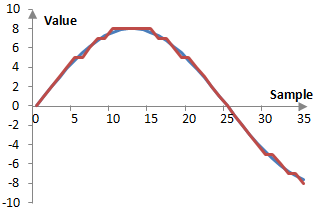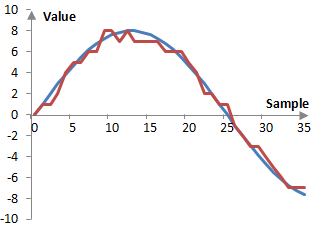# Dithering

Dithering is the process of introducing random noise into a signal to remove errors that are correlated to the signal.

When a signal is recorded digitally (a process sometimes called quantization) and as in the example below, there is a potential for introducing systematic errors. In a periodic signal, the errors will be repeated with the same periodicity as the signal and will be a harmonic distortion of the signal. Due to limitations in digital formats, these errors are unavoidable. Since these errors cannot be avoided, by introducing random noise (dithering), these errors are made non-systematic.

## Quantization errors

CD quality audio, for example, uses 16-bit signed integer representation of the signal. The values of the signal are recorded with the integer numbers between -32768 and 32768. If the true value of the signal is not an integer number, it would be rounded (up or down) or simply truncated (down) before it is recorded. Thus, CD quality audio ultimately contains rounded or truncated data. Since such truncation is difficult to examine visually with the 44100 Hz 16-bit representation of the CD, consider the following example of a 2 Hz wave (blue) sampled at the sampling rate of 100 Hz and encoded with the 4-bit sampling resolution (red). Although the true value of the wave at sample 5 is 4.702, it has been recorded as 5. Although its true value at sample 6 is 5.476, it has also been recorded as 5. It is impossible to record the wave at its true values with the given sampling resolution of 4 bits.Although experts may argue whether such quantization errors are audible in an audio recording (quantization errors are very obvious in some digital images, such as GIF files, where the color resolution allows only 256 colors), it is clear that such errors are systematic, quantifiable, and unavoidable. If the signal in the figure above repeats indefinitely, the error will also repeat indefinitely, in exactly the same fashion. This kind of error then is periodic with the same periodicity as the signal and is correlated to the signal. In other words, the error is a harmonic distortion of the signal.

Note that, since these errors are small, if they are audible, it is more likely that they would be audible in an audio signal recorded at low levels, when the levels of the audio signal are raised. Finally, note that the errors on the figure above are specific to the sampling frequency, bit resolution, and signal. Different errors may manifest themselves with different signals, different sampling frequencies, or different sampling resolutions.

## Dithering

Since quantization errors are unavoidable, the best thing to do is to make them non-systematic. Non-systematic errors would not be a harmonic distortion to the signal. We do so by introducing random noise in the signal of similar magnitude to the error.

While dithering does not remove errors, it at least ensures that such errors are no longer correlated to the signal. If we introduce dithering to the signal in the figure above, we might get something similar to figure below.This figure is the result of randomly choosing whether to round up or down each value of the wave. This is equivalent to adding a random number between -0.5 and 0.5 to the wave and then rounding (up or down). This is the simplest type of dithering – one that uses a uniform distribution of the random change to the error and is done for every sample (at the sampling frequency).

There are several ways in which the dither can be changed. First, this dither above uses a uniform distribution of the added error and hence it is said to have a rectangular probability density function – it is an RPDF dither. Common dithers in audio processing are the RPDF dither and the triangular probability density function or TPDF dither. RPDF dither is said to make the first moment of the error uncorrelated and TPDF is said to make the first and second moments of the error uncorrelated. Other probability density functions are also possible.

The dither can also be changed by introducing errors with a different frequency, which is usually known as dither coloration. The purpose of this is typically to dither with frequencies that are outside of the range, where the ear is most sensitive, say, outside of 1 KHz to 5 KHz (although we should note that the threshold of hearing curves – Fletcher-Munson curves – is always dependent on the signal level and there is no interval of ear sensitivity that is independent of the level of the signal). This can be done by simply filtering the random dither and is also sometimes called noise shaping, although there is a differences between dither coloration and noise shaping. Noise shaping can be applied to reshape the inherent quantization errors without introducing dither at all.

Finally, we can modify the amplitude of the dither. The dithered signal in the figure above changes the original quantized signal by adding a bit at random samples, subtracting a bit, or leaving the bit representation of the signal unchanged. In other words, dithering works by changing the lowest – least significant – bits of the signal and is thought of as a least significant bit or LSB operation. It is typical to use noise of one or two of the least significant bits.

## Using dithers

Dithering should not be over-used and should not be used arbitrarily. First, there are no quantization errors when there is no signal. One should not introduce random noise if there is no systematic noise, such as between audio tracks. Alternatively, a dither should be designed in such a way that it settles at zero when the signal settles at zero. Finally, dithering should not be applied to signals which are subject to further processing, since there may be interactions between the dither and quantization or dithers applied later.

### Filtered HTML

• Freelinking helps you easily create HTML links. Links take the form of [[indicator:target|Title]]. By default (no indicator): Click to view a local node.